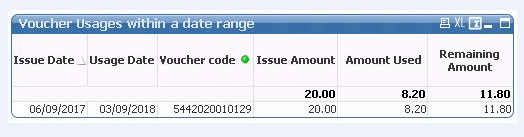# QlikView App Dev

Discussion Board for collaboration related to QlikView App Development.

Announcements
QlikWorld 2023, a live, in-person thrill ride. Save \$300 before February 6: REGISTER NOW!
cancel
Showing results for
Did you mean:Partner - Creator

## Problem with set analysis in sum function

Hi all,

I have a chart which displays a list of vouchers and their usage. KPIs include : issue amount, amount used and remaining amount.

The goal is to display a list of vouchers used within a period of dates. Attached is an image which represents the underlaying data and the chart.

KPI expressions:

Issue amount = sum({<[Usage date]={">=\$(v_UsageDateStart) <=\$(v_UsageDateEnd)"}>} Issue Amount)

Amount usedsum({<[Usage date]={">=\$(v_UsageDateStart) <=\$(v_UsageDateEnd)"}>} Amount Used)

Remaining Amount = Issue amount  - Amount used

The problem is with the "Amount Used" because it takes into account only usages occuring within the date selection.  The client wants that it includes all usages per voucher occuring until the \$(v_UsageDateEnd).

I tried using TOTAL in the expression but it's not working (same result as previously)

Amount used = sum(TOTAL<[Voucher Code]> {<[Usage date]={">=\$(v_UsageDateStart) <=\$(v_UsageDateEnd)"}>} Amount Used)

The following is not working either because it modifies the perimeter of the search :

Amount used = sum({<[Usage date]={"<=\$(v_UsageDateEnd)"}>} Amount Used)

I was thinking about Aggr() function but not sure how to implement it.

1 Solution

Accepted SolutionsMVP

```If(Column(1) <> 0,
RangeSum(
Sum({<[Usage date] = {">=\$(v_UsageDateStart)<=\$(v_UsageDateEnd)"}>} [Issue Amount]),
-Sum(TOTAL <[Voucher Code]> {<[Usage date] = {"<=\$(v_UsageDateEnd)"}>} [Amount Used])
))```
17 RepliesMVP

So, for Amount Used, you want to see 4.7 + 35 for Voucher Code 111?Partner - Creator
Author

This is the idea but only for KPI Remaining Amount.

For voucher 111 we should have :

Used Amount (only within the search periode) = 4.7

Remaining Amount = 10.3  (50 - 35 - 4.7)MVP

So, this should work... I think

```RangeSum(
Sum({<[Usage date] = {">=\$(v_UsageDateStart)<=\$(v_UsageDateEnd)"}>} [Issue Amount]),
-Sum({<[Usage date] = {"<=\$(v_UsageDateEnd)"}>} [Amount Used])
)```Partner - Creator
Author

I tried this expression but then all usages are displayed (even those outside the date range).MVP

Can you share a screenshot of when you use this expression?Partner - Creator
Author

Here it is.MVP

May be do this

```If(Column(1) <> 0,
RangeSum(
Sum({<[Usage date] = {">=\$(v_UsageDateStart)<=\$(v_UsageDateEnd)"}>} [Issue Amount]),
-Sum({<[Usage date] = {"<=\$(v_UsageDateEnd)"}>} [Amount Used])
))```Partner - Creator
Author

Now there is only the usage within the date range but remaining amount should be 0 (image attached).MVP

I don't think I understand the problem with the most recent image... what is wrong here?Community Browser